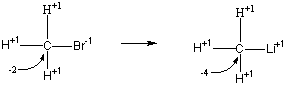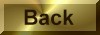Practice Problem 8

Methyllithium (CH3Li) can be used to form bonds between carbon and either main-group metals or transition metals:

HgCl2(s) + 2 CH3Li(l)Hg(CH3)2(l) + 2 LiCl(s)

WCl6(s) +  6 CH3Li(l)W(CH3)6(l) + 6 LiCl(s)

It can be used also to form bonds between carbon and other nonmetals:

PCl3(s) +  3 CH3Li(l)P(CH3)3(l) + 3 LiCl(s)

or between carbon atoms:

CH3Li(l) + H2CO(g)[CH3CH2OLi]CH3CH2OH(l)

Use Lewis structures to explain the stoichiometry of the following oxidation-reaction, which is used to synthesize methyllithium:

CH3Br(l) + 2 Li(s)CH3Li(l) + LiBr(s)

Answer

The active metals are reducing agents in all of their chemical reactions.  In this reaction, lithium metal reduces a carbon atom from an oxidation state of -2 to -4, as shown below:

Reduction: CH3Br + 2 e-CH3LiThe other half of the reaction therefore must involve the net loss of a total of two electrons:

Oxidation: 2 Li2 Li+ + 2 e-

Two moles of lithium are therefore consumed for each mole of CH3Br in this reaction.  One of the Li+ ions formed in this reaction combines with the negatively charged carbon atom to form methyllithium and the other participates from solution with the Br - ion as LiBr:

CH3Br(l) + 2 Li(s)CH3Li(l) + LiBr(s)